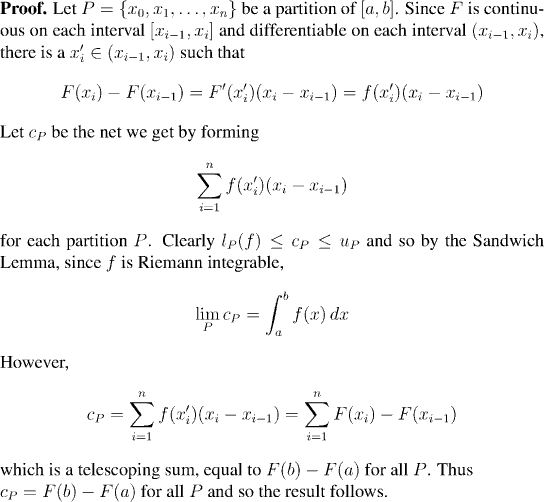Analysis WebNotes
arrow_backThe key ingredient in this proof is the Mean Value Theorem. This ought not to be surprising; the Mean Value Theorem is the tool we always use to pass from information about the derivative of a function at points (``local information'') to deduce facts about the behavior of the function as a whole (``global information''). For example, it was the Mean Value Theorem which we already used to prove that when the derivative is positive, then the function is increasing.